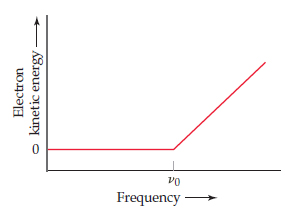# Problem: In an experiment to study the photoelectric effect, a scientist measures the kinetic energy of ejected electrons as a function of the frequency of radiation hitting a metal surface. She obtains the following plot.The point labeled "v0" corresponds to light with a wavelength of 536 nm. What is the value of v0 in s–1?

⚠️Our tutors found the solution shown to be helpful for the problem you're searching for. We don't have the exact solution yet.

###### Problem Details

In an experiment to study the photoelectric effect, a scientist measures the kinetic energy of ejected electrons as a function of the frequency of radiation hitting a metal surface. She obtains the following plot.The point labeled "v0" corresponds to light with a wavelength of 536 nm. What is the value of v0 in s–1?